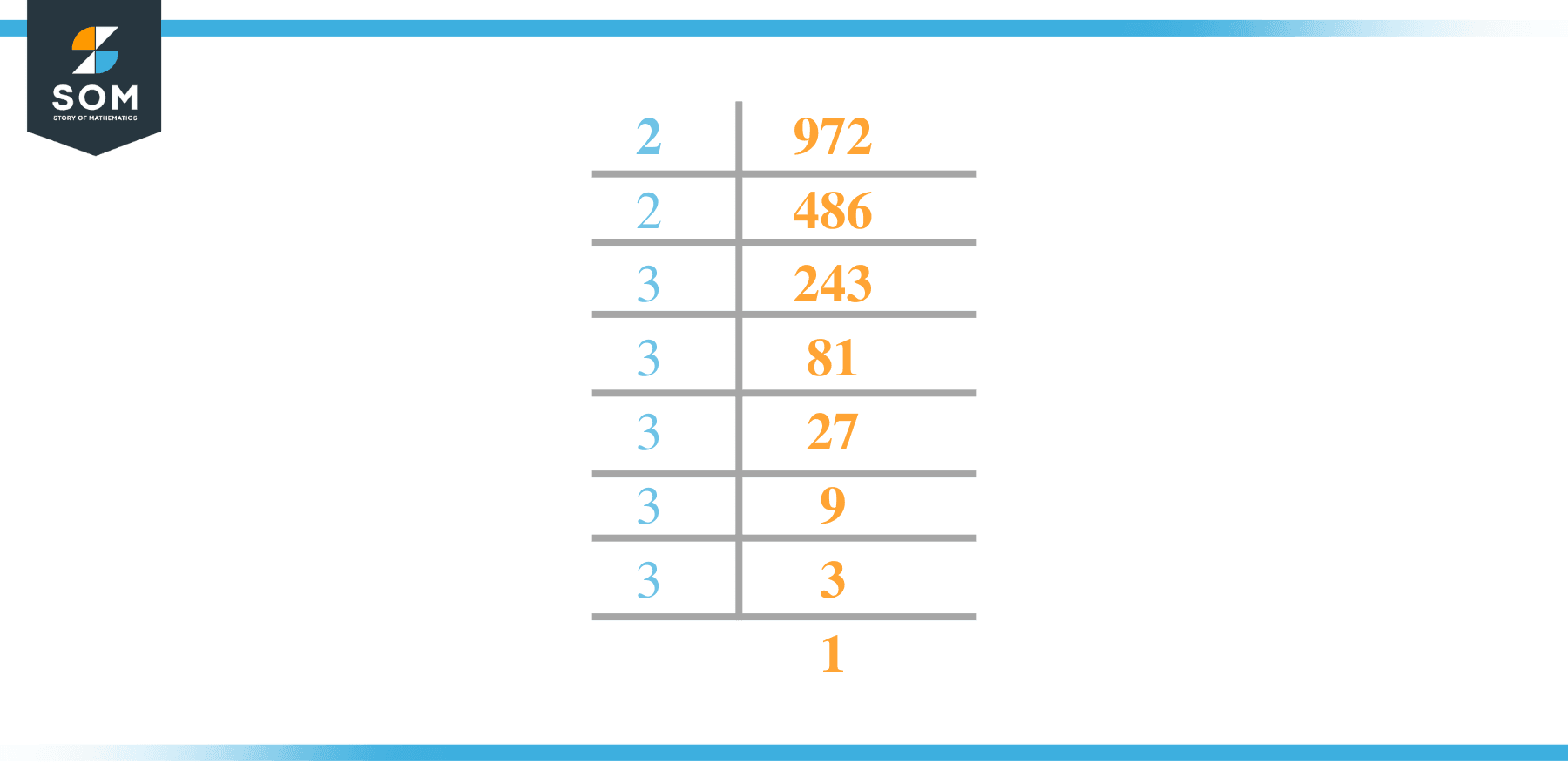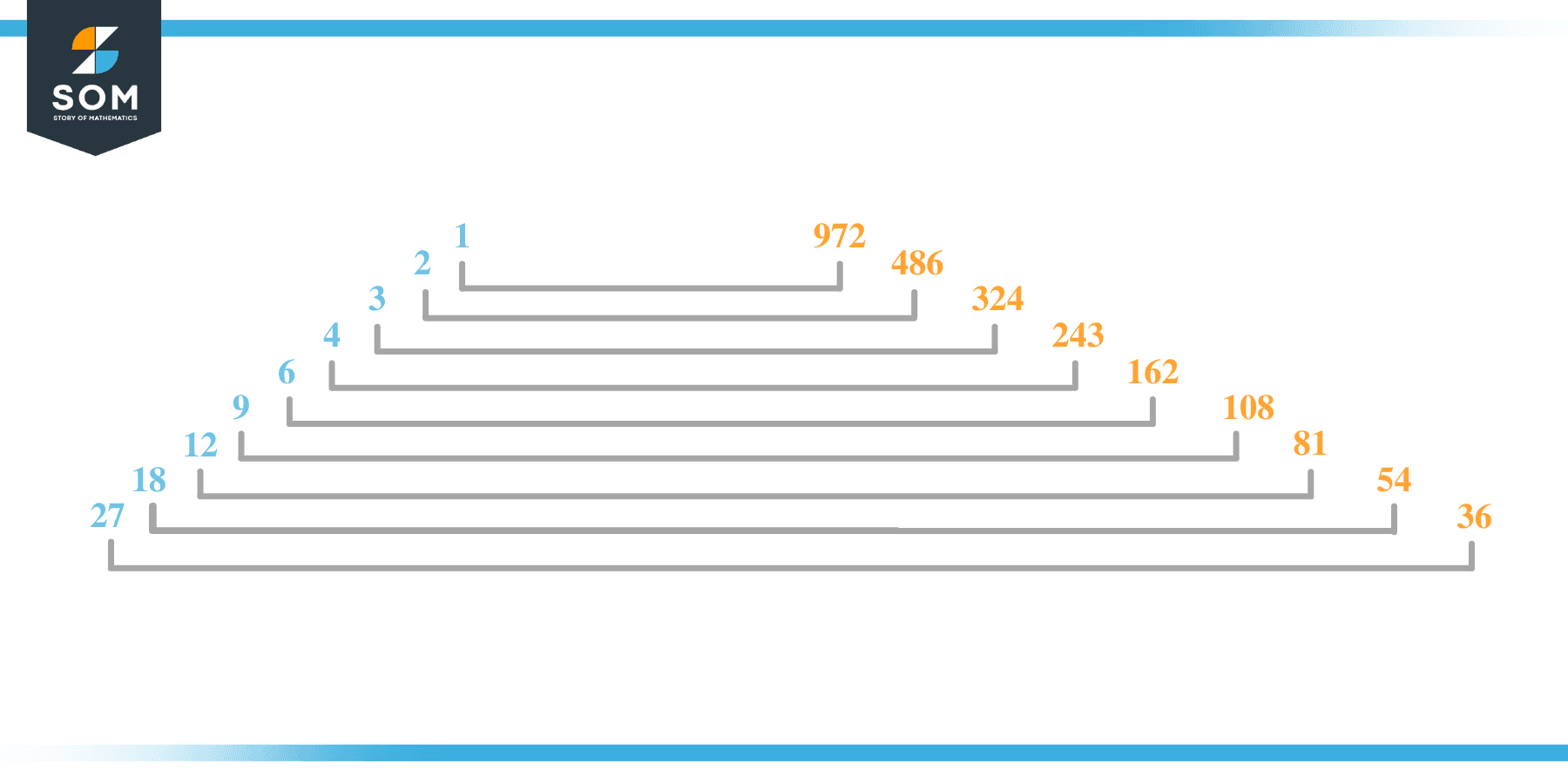# Factors of 972: Prime Factorization, Methods, and Examples

The factors of 972 are a set of particular numbers that do not leave any remainder when divided by 972. It is a composite number having 18 factors in total.The factors can be positive or negative depending upon the integers multiplied to obtain the given number.

### Factors of 972

Here are the factors of number 972.

Factors of 972: 1, 2, 3, 4, 6, 9, 12, 18, 27, 36, 54, 81, 108, 162, 243, 324, 486, 972

### Negative Factors of 972

The negative factors of 972 are similar to their positive aspects, just with a negative sign.

Negative Factors of 972: -1, -2, -3, -4, -6, -9, -12, -18, -27, -36, -54, -81, -108, -162, -243, -324, -486, and -972

### Prime Factorization of 972

The prime factorization of 972 is the way of expressing its prime factors in the product form.

Prime Factorization: 2 x 2 x 3 x 3 x 3 x 3 x 3

In this article, we will learn about the factors of 972 and how to find them using various techniques such as upside-down division, prime factorization, and factor tree.

## What Are the Factors of 972?

The factors of 972 are 1, 2, 3, 4, 6, 9, 12, 18, 27, 36, 54, 81, 108, 162, 243, 324, 486, and 972. These numbers are the factors as they do not leave any remainder when divided by 972.

The factors of 972 are classified as prime numbers and composite numbers. The prime factors of the number 972 can be determined using the prime factorization technique.

## How To Find the Factors of 972?

You can find the factors of 972 by using the rules of divisibility. The divisibility rule states that any number, when divided by any other natural number, is said to be divisible by the number if the quotient is the whole number and the resulting remainder is zero.

To find the factors of 972, create a list containing the numbers that are exactly divisible by 972 with zero remainders. One important thing to note is that 1 and 972 are the 972’s factors as every natural number has 1 and the number itself as its factor.

1 is also called the universal factor of every number. The factors of 972 are determined as follows:

$\dfrac{972}{1} = 972$

$\dfrac{972}{2} = 486$

$\dfrac{972}{3} = 324$

$\dfrac{972}{4} = 243$

$\dfrac{972}{6} = 162$

$\dfrac{972}{9} = 108$

$\dfrac{972}{12} = 81$

$\dfrac{972}{18} = 54$

$\dfrac{972}{27} = 36$

$\dfrac{972}{972} = 1$

Therefore, 1, 2, 3, 4, 6, 9, 12, 18, 27, 36, 54, 81, 108, 162, 243, 324, 486, and 972 are the factors of 972.

### Total Number of Factors of 972

For 972, there are 18 positive factors and 18 negative ones. So in total, there are 36 factors of 972.

To find the total number of factors of the given number, follow the procedure mentioned below:

1. Find the factorization/prime factorization of the given number.
2. Demonstrate the prime factorization of the number in the form of exponent form.
3. Add 1 to each of the exponents of the prime factor.
4. Now, multiply the resulting exponents together. This obtained product is equivalent to the total number of factors of the given number.

By following this procedure, the total number of factors of 972 is given as:

Factorization of 972 is 1 x 2 x 2 x 3 x 3 x 3 x 3 x 3.

The exponent of 1, 2, and 3 is 1, 2, and 5 respectively.

Adding 1 to each and multiplying them together results in 36.

Therefore, the total number of factors of 972 is 36. 18 is positive, and 18 factors are negative.

### Important Notes

Here are some essential points that must be considered while finding the factors of any given number:

• The factor of any given number must be a whole number.
• The factors of the number cannot be in the form of decimals or fractions.
• Factors can be positive as well as negative.
• Negative factors are the additive inverse of the positive factors of a given number.
• The factor of a number cannot be greater than that number.
• Every even number has 2 as its prime factor, the smallest prime factor.

## Factors of 972 by Prime FactorizationThe number 972 is a composite number. Prime factorization is a valuable technique for finding the number’s prime factors and expressing the number as the product of its prime factors.

Before finding the factors of 972 using prime factorization, let us find out what prime factors are. Prime factors are the factors of any given number that are only divisible by 1 and themselves.

To start the prime factorization of 972, start dividing by its most minor prime factor. First, determine that the given number is either even or odd. If it is an even number, then 2 will be the smallest prime factor.

Continue splitting the quotient obtained until 1 is received as the quotient. The prime factorization of 972 can be expressed as:

972 = 2 x 2 x 3 x 3 x 3 x 3 x 3

## Factors of 972 in PairsThe factor pairs are the duplet of numbers that, when multiplied together, result in the factorized number. Factor pairs can be more than one depending on the total number of factors given.

For 972, the factor pairs can be found as:

1 x 972 = 972

2 x 486= 972

3 x 324 = 972

4 x 243 = 972

6 x 162 = 972

9 x 108 = 972

12 x 81 = 972

18 x 54 = 972

27 x 36 = 972

The possible factor pairs of 972  are given as (1, 972), (2, 486), (3, 324), (4, 243), (6, 162), (9, 108), (12, 81), (18, 54) and (27, 36).

All these numbers in pairs, when multiplied, give 972 as the product.

The negative factor pairs of 972 are given as:

-1 x -972 = 972

-2 x -486= 972

-3 x -324 = 972

-4 x -243 = 972

-6 x -162 = 972

-9 x -108 = 972

-12 x -81 = 972

-18 x -54 = 972

-27 x -36 = 972

It is important to note that in negative factor pairs, the minus sign has been multiplied by the minus sign, due to which the resulting product is the original positive number. Therefore, -1, -2, -3, -4, -6, -9, -12, -18, -27, -36, -54, -81, -108, -162, -243, -324, -486, and -972 are called negative factors of 972.

The list of all the factors of 972, including positive as well as negative numbers, is given below.

Factor list of 972: 1, -1, 2, -2, 3, -3, 4, -4, 6, -6, 9, -9, 12, -12, 18, -18, 27, -27, 36, -36, 54, -54, 81, -81, 108, -108, 162, -162, 243, -243, 324, -324, 486, -486, 972, and -972

## Factors of 972 Solved Examples

To better understand the concept of factors, let’s solve some examples.

### Example 1

How many factors of 972 are there?

### Solution

The total number of Factors of 972 is 36.

Factors of 972 are 1, 2, 3, 4, 6, 9, 12, 18, 27, 36, 54, 81, 108, 162, 243, 324, 486, and 972.

### Example 2

Find the factors of 972 using prime factorization.

### Solution

The prime factorization of 972 is given as:

972 $\div$ 2 = 486

486 $\div$ 2 = 243

243 $\div$ 3 = 81

81 $\div$ 3 = 27

27 $\div$ 3 = 9

9 $\div$ 3 = 3

3 $\div$ 3 = 1

So the prime factorization of 972 can be written as:

2 x 2 x 3 x 3 x 3 x 3 x 3 = 972